Next: Fit Quality Up: Analysis Previous: Fitting Software

# Calculation of the Core Radius

Substituting the fitted parameters into expression (5.2) reveals the spatial profile of the internal field B(r) within the sample. Figure 5.3 displays the profile around a flux line generated by an external field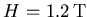at temperature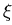.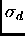The inset contains the internal field distribution n(B) belonging to this spatial profile and, for comparison, the real amplitude of the FFT of the recorded precession signal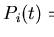. The two distributions are similar; however, as alluded to in Section 5.1, the finite time span of the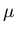SR data broadens the FFT and creates rapid oscillations in it. This ringing is especially visible in the high field B tail of the FFT, despite the FFT having undergone apodisation to smooth it. Apodisation effectively convolutes the FFT with a Gaussian function, and so broadens the distribution still further. Nuclear dipolar fields and slight vortex lattice disorder also broaden this distribution. The small peak in the FFT at fieldarises from muons that miss the sample. The maximum peak in the FFT, and in the fitted field distribution n(B), occurs for the field B located at the midpoint between nearest neighbour vortices. The shoulder corresponding to fields B weaker than this, expected for a square vortex lattice according to the local London model, is absent here in both the fitted field distribution n(B) and the FFT of the recorded polarisation. This lack of a sizeable low field shoulder is a consequence of nonlocality. The effect of nonlocality on the field distribution n(B) appears in more detail in the next chapter. The very high fields B in the field distribution n(B) of a flux line lattice derive from the vortex core region.

A useful definition of the radius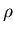of a vortex core is the distance r from the core centre to the point where the supercurrent density J(r) is greatest. Applying one of Maxwell's equations,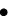(7.7)

where the constantis the permeability of the medium, to the fitted internal field B(r) readily supplies the supercurrent density J(r). Figure 5.4 depicts the computed supercurrent density J(r) along a straight line between nearest neighbour vortices, and the extracted core radius, due to an external fieldat temperature.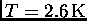The supercurrent density J(r) grows from zero at the vortex centre to its maximum value at the core radius, and then subsides until it vanishes at the midpoint between adjacent vortices. All core radius values presented in this thesis are deduced from the fitted field  B(r) in this way. Core radii determined by these means are relatively insensitive, compared to the fitted parameters, to the specific choice of internal field model, as long as the model reproduces the actual field of the vortex lattice reasonably well . This is because the calculated supercurrent density J(r), and hence the core radius, depend only on the spatial profile of the internal field  B(r). The accuracy to which the model reconstructs the field  B(r) can be inferred from the quality of the fit between the measured muon precession signaland that predicted by the model.Next: Fit Quality Up: Analysis Previous: Fitting Software
Jess H. Brewer
2001-10-31